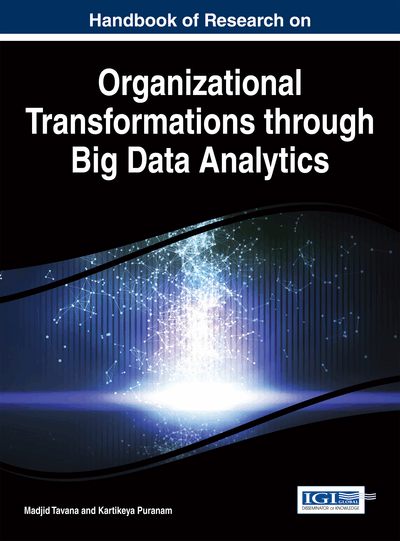# Some Aspects of Estimators for Variance of Normally Distributed Data

N. Hemachandra (Indian Institute of Technology Bombay, India) and Puja Sahu (Indian Institute of Technology Bombay, India)
DOI: 10.4018/978-1-4666-7272-7.ch021
Available
\$29.50
No Current Special Offers

## Abstract

Normally distributed data arises in various contexts and often one is interested in estimating its variance. The authors limit themselves in this chapter to the class of estimators that are (positive) multiples of sample variances. Two important qualities of estimators are bias and variance, which respectively capture the estimator's accuracy and precision. Apart from the two classical estimators for variance, they also consider the one that minimizes the Mean Square Error (MSE) and another that minimizes the maximum of the square of the bias and variance, the minmax estimator. This minmax estimator can be identified as a fixed point of a suitable function. For moderate to large sample sizes, the authors argue that all these estimators have the same order of MSE. However, they differ in the contribution of bias to their MSE. The authors also consider their Pareto efficiency in squared bias versus variance space. All the above estimators are non-dominated (i.e., they lie on the Pareto frontier).
Chapter Preview
Top

## 1. Introduction

It is well known that normally distributed data sets are observed in numerous situations. One major reason for this is the following situation. Suppose one has a random error which is the aggregate of a large collection of errors. Then, under mild conditions, by the classical Central Limit Theorem and its variants, as discussed in Billingsley (1995), Chung (2001), Fristedt and Gray (1997), Kallenberg (2002), Wasserman (2005), etc., the standardized sum of the collections of errors (and hence suitable scaling of the centered random error) has approximately the distribution of a zero mean normal random variable.

A central theme in statistical inference is that given a sample from a parametric distribution, one is interested in finding a suitable ‘best’ estimator for a parameter of the distribution. Most of such inference procedures concentrate on the unbiased estimators and finding the ‘best’ (i.e., the one having the minimum variance) amongst them. These are the classical Uniformly Minimum Variance Unbiased Estimators, (UMVUE) (Casella & Berger, 2002), (DeGroot & Schervish, 2012), etc. However, one can tradeoff the bias of the estimator to achieve lower variance and hence find a better estimator in terms of Mean Squared Error (MSE) as MSE is the sum of squared bias and variance of the estimator, leading to the optimal MSE estimator. Further, one can view both squared bias and variance of an estimator as equally important and hence search for an estimator that minimizes the maximum of these two (undesirable) quantities, the minmax estimator.

In fact, one can view MSE as capturing a quality of an estimator and hence compare various estimators on the basis of their MSEs. Also, one can compare estimators in terms of the percentage of squared bias in MSE. Yet an another way to compare estimators is to view this comparison as a multi-criteria problem involving squared bias and variance and then search for those estimators that are Pareto optimal: the set of estimators such that reducing of one of these quantities leads to increase in the other quantity.

In this chapter, we illustrate the above aspects of estimators and various measures of quality of estimators when the underlying data is normally distributed and the parameter we are interested is the variance of this normal random variable.

Consider a random sample (X1, X2, ..., Xn) of sizefrom a N(µ,σ2) distribution and consider the two cases for estimation of population variance (σ2): µ known and µ unknown. For µ known case, the classical unbiased estimator is

(1)

But one can also consider the following estimators of σ2:

, (2) parameterized by coefficients,.can be viewed as scalings of.

Similarly for the µ unknown case, we decided to look for estimators for σ2 of the form2,. (3)

It is assumed that the sample size,, is at least two. Also, we can restrict ourselves toas estimators of this nature dominate the zero estimator corresponding to, both on MSE as well as minmax criteria. Details on this and related points are in given in the technical report (Hemachandra & Sahu, 2014).

## Key Terms in this Chapter

Minmax Estimator: We define the minmax estimator as the one which minimizes the maximum of the squared bias and the variance values for an estimator with coefficient, c . .

Pareto Optimality: Pareto optimality or efficiency is achieved over the multi-criteria for an estimator, when none of the component can be improved upon without making worse the other component.

Variance: The variance of an estimator T of the parameter ? is defined as: .

Bias: The bias of an estimator T of the parameter ? is defined as: .

MSE: Mean Squared Error (MSE) of an estimator T of the parameter ? is defined as: , which can be simplified to the following form: .

## Complete Chapter List

Search this Book:
Reset